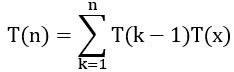October 8, 2023
October 8, 2023
October 8, 2023
###### Data-Structures
October 8, 2023
 Question 49

Let T(n) be the number of different binary search trees on n distinct elements. Then, where x is

 A n – k + 1 B n – k C n – k – 1 D n – k – 2
Question 49 Explanation:
A binary search tree consists of n distinct elements. Let consider on left subtree, it consists of (k-1) elements. Then right subtree consists of (n-k) elements. From this we c an write recursive function as T(k-1)*(n-k) i.e.,Question 49 Explanation:
A binary search tree consists of n distinct elements. Let consider on left subtree, it consists of (k-1) elements. Then right subtree consists of (n-k) elements. From this we c an write recursive function as T(k-1)*(n-k) i.e.,